Mechanical Engineering

Design and simulate your mechanical systems as interactive applications using built-in surface-modeling capabilities, the finite element method, control systems, and sophisticated optimization routines—one system, one integrated workflow.

Underlying the Wolfram mechanical engineering solution is the world's most accurate symbolic and numeric engine, with highly automated superfunctions for differential equation solving and large-scale eigensystem computation, all with self-checking high-precision arithmetic.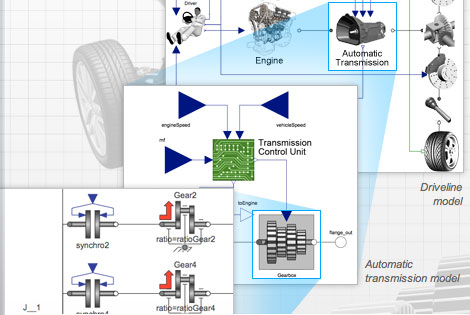Analyzing a drive cycle with Wolfram SystemModeler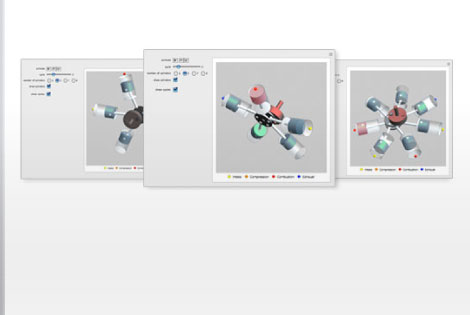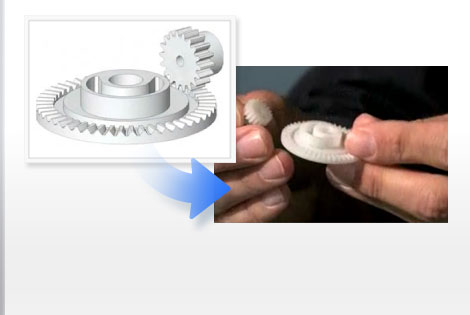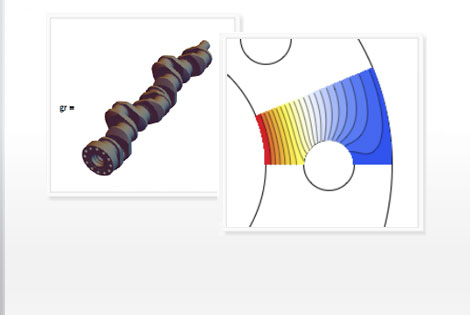The Wolfram Edge
How Wolfram Compares
Key Capabilities

Wolfram technologies include thousands of built-in functions that let you:

• Design and simulate powertrain controllers, heavy equipment, vehicle dynamics, space robotics, and more with Wolfram SystemModeler
• Automatically compute design quantities, including closed-loop transfer functions, PID parametrizations, and more
• Perform automated finite element analysis, including grid and element generation
• Model real-world systems that include parts from multiple physical domains, such as mechanics, electronics, and control systems, with Wolfram SystemModeler
• Calculate free and forced vibrations of linear damped, lumped-parameter, multi-degree-of-freedom models of mechanical systems
• Optimize the design parameters of mechanical systems like rack-and-gear mechanisms, piston-crankshaft assembly, and more
• Develop speed and torque control algorithms for motor, servo, and inverter drives
• Predict failure of components
• Perform surface modeling of complex surfaces, including automobile bodies, gas turbine blades, medical equipment, and more
• Use built-in functions for Taylor series and Fourier series analysis for applications in vibrations, system dynamics, and more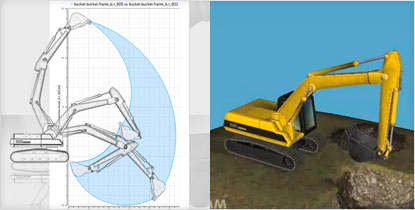Designing, analyzing, and simulating the reachable positions of an excavator bucket with Wolfram SystemModeler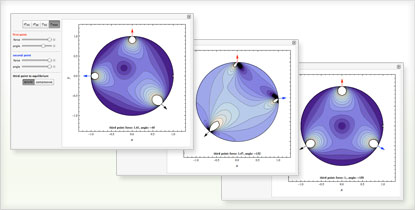The Wolfram Edge
How Wolfram Compares
Key Capabilities

• Free-form linguistic input produces immediate results without the need for syntax
Unique to Wolfram technologies
• Built-in industrial-strength surface-modeling primitives for modeling highly customized surfaces, such as medical implant devices, automobile bodies, and more
MATLAB does not have built-in surface-modeling primitives
• Highly optimized superfunctions analyze your equations and automatically select the right algorithms to get you accurate results quickly—sometimes switching mid-calculation for further optimization
Other computation systems make you analyze your equations manually to determine which function to apply—e.g., where in Mathematica you use NDSolve, in MATLAB you must correctly choose among ode45, ode23, ode113, ode15s, bvp4c, pdepe, and so on or risk wrong answers
• Analyze and optimize mechanical assemblies in one system, using built-in constrained and unconstrained optimization routines
MATLAB requires an extra-cost toolbox for optimization; Pro/Engineer requires the extra-cost Pro/Mechanica add-on for design optimization
• Automated precision control and arbitrary-precision numerics produce accurate results for large-scale finite element analysis problems
MATLAB relies on finite-precision numerics, which can cause serious errors due to lack of precision
• Automatically compute higher-order differential equations using Mathematica's mixed symbolic-numeric architecture
MATLAB requires you to manually rewrite higher-order differential equations into first-order equations for computation
• Instantly build interactive applications to prototype dynamic systems
Unique to Wolfram technologies
• Write functions that generate other functions using Mathematica's functional programming capabilities for nonstandard applications, such as creating FEA code that is independent of space dimensions
MATLAB and other procedural languages do not support this functionality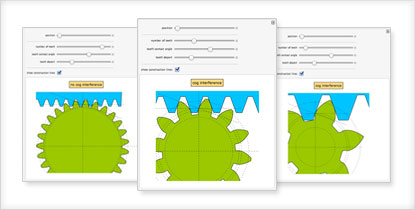Interactively exploring the effects of changing involute gear parameters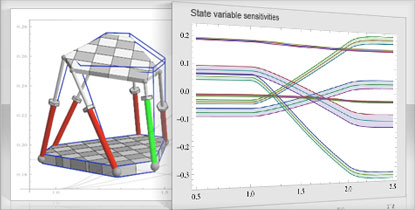Performing sensitivity analysis on a Stewart platform

The Wolfram Edge
How Wolfram Compares
Key Capabilities

Wolfram SystemModeler is the most complete physical modeling and simulation tool for high-fidelity modeling. With SystemModeler, you can: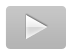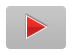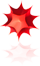Get Mathematica

System requirements »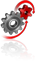Get SystemModeler

System requirements »